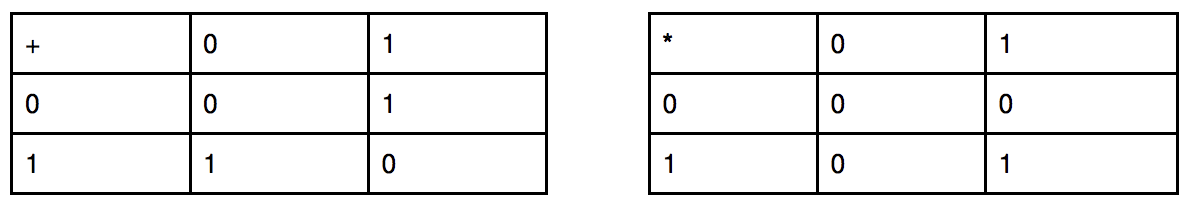시간 제한메모리 제한제출정답맞힌 사람정답 비율
1 초 256 MB20161684.211%

## 문제

Harry Potter has found another strange spell in Half-blood Prince diary, that could generate a different binary vector of size M. As he is not the best magician, this spell does not work perfectly so he could generate only vectors where exactly 2 elements are non zero. Harry has used this spell N times and he has constructed a matrix of M rows and N columns, where all generated vectors are columns.

Now Harry has a class of Magical Matrix Theory, where the professor asked him to calculate the rank of such a matrix. You are here to help him!

Operations in Magical Matrix Theory satisfied next rules:The rank of a matrix A corresponds to the maximal number of linearly independent columns of A. The vectors in a set $$T = \{\vec{v_1}, \vec{v_2}, \dots, \vec{v_k}\}$$ are said to be linearly independent if the equation $$a_1\vec{v_1} + a_2\vec{v_2} + \dots + a_k\vec{v_k} = \vec{0}$$, where $$a_i = \{0, 1\}$$ for $$i = 1, \dots, k$$ can only be satisfied by $$a_i = 0$$ for $$i = 1, \dots, k$$.

## 입력

On the first line two integers - M (size of vectors) and N (number of vectors generated by Harry). Each of the next M lines has the format: ki, c1, c2, ..., cki, where ki is the number of non-zero elements in row i. The next ki numbers are column indexes (1 ≤ cj ≤ N, j = 1, ..., ki), which are non-zero in this row. For more details, see exampels.

• 1 ≤ N ≤ 105
• 2 ≤ M ≤ 105
• 0 ≤ ki ≤ N

## 예제 입력 1

3 3
2 1 3
2 1 2
2 2 3


## 예제 출력 1

2


## 예제 입력 2

4 3
3 1 2 3
1 1
1 2
1 3


## 예제 출력 2

3


## 힌트

In first example, Harry has generated 3 vectors:

$$\vec{v_1} = (1, 1, 0), \vec{v_2} = (0, 1, 1), \vec{v_3} = (1, 0, 1)$$

and the matrix is:

\begin{bmatrix} 1 & 0 & 1 \\ 1 & 1 & 0 \\ 0 & 1 & 1 \end{bmatrix}

But $$\vec{v_1} + \vec{v_2} + \vec{v_3} = \vec{0}$$.